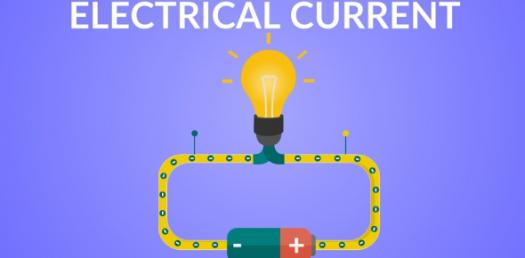# Physics Quiz: Current Electricity Trivia Questions!

10 Questions | Total Attempts: 3282SettingsRelated Topics
• 1.
Charge of 60 C passes through an electric lamp in 2 minutes. Then the current in the lamp is
• A.

0.5 A

• B.

30 A

• C.

1 A

• D.

5A

• 2.
The material through which electric charge can flow easily is
• A.

Copper

• B.

Germanium

• C.

Quartz

• D.

Mica

• 3.
The current flowing in a conductor is proportional to
• A.

Drift velocity

• B.

1/ area of cross section

• C.

1/no of electrons

• D.

Square of area of cross section.

• 4.
A toaster operating at 240V has a resistance of 120Ω. The power is
• A.

480 W

• B.

400 W

• C.

2 W

• D.

240 W

• 5.
If the length of a copper wire has a certain resistance R, then on doubling the length its specific resistance
• A.

Will remain the same

• B.

Will be doubled

• C.

Will become 1/4 th

• D.

Will become 4 times

• 6.
• A.

1 Ω

• B.

2 Ω

• C.

0.5 Ω

• D.

4 Ω

• 7.
In the case of insulators, as the temperature decreases, resistivity
• A.

Increases

• B.

Becomes zero

• C.

Decreases

• D.

Remains constant

• 8.
If the resistance of a coil is 2 Ω at and α = 0.004 /, then its resistance at  is
• A.

2.8 Ω

• B.

0 Ω

• C.

1.4 Ω

• D.

4 Ω

• 9.
According to Faraday’s law of electrolysis, when a current is passed, the mass of ions deposited at the cathode is independent of
• A.

Resistance

• B.

Time

• C.

Charge

• D.

Current

• 10.
When n resistors of equal resistances (R) are connected in series, the effective resistance is
• A.

NR

• B.

1/nR

• C.

R/n

• D.

N/R